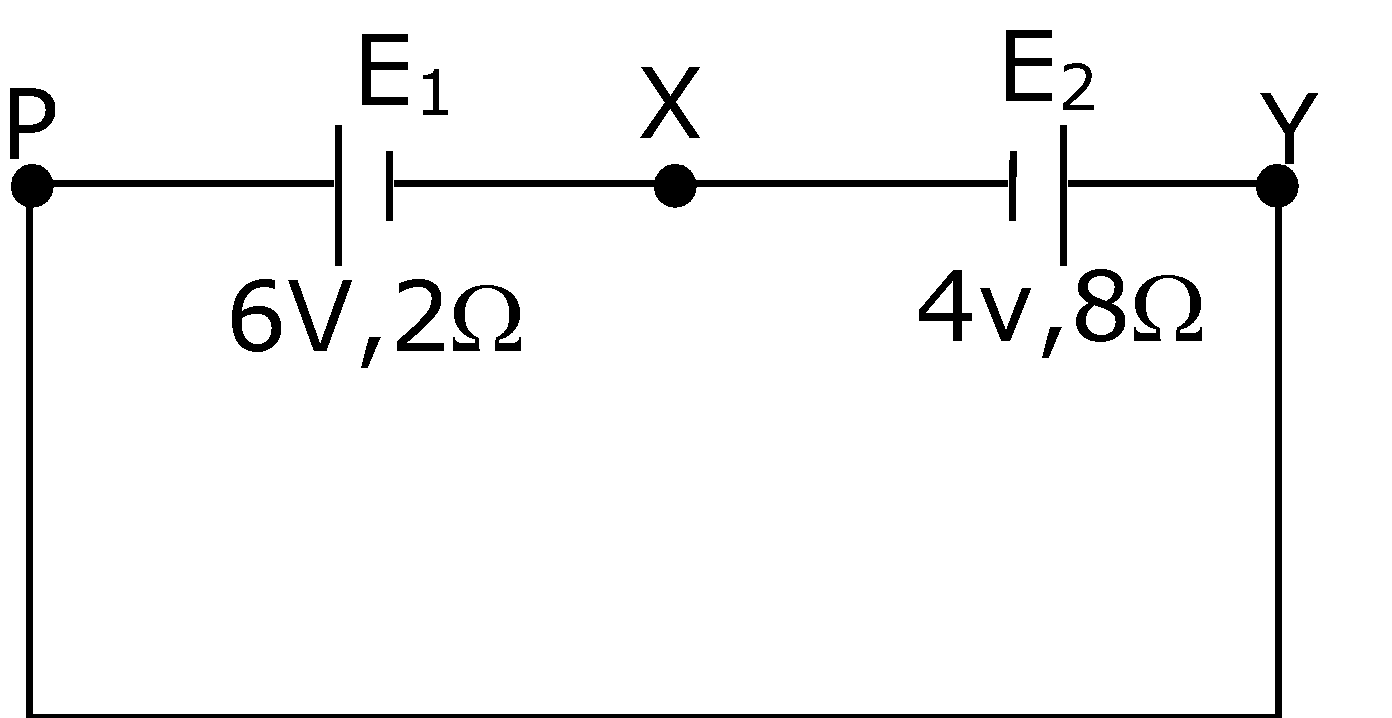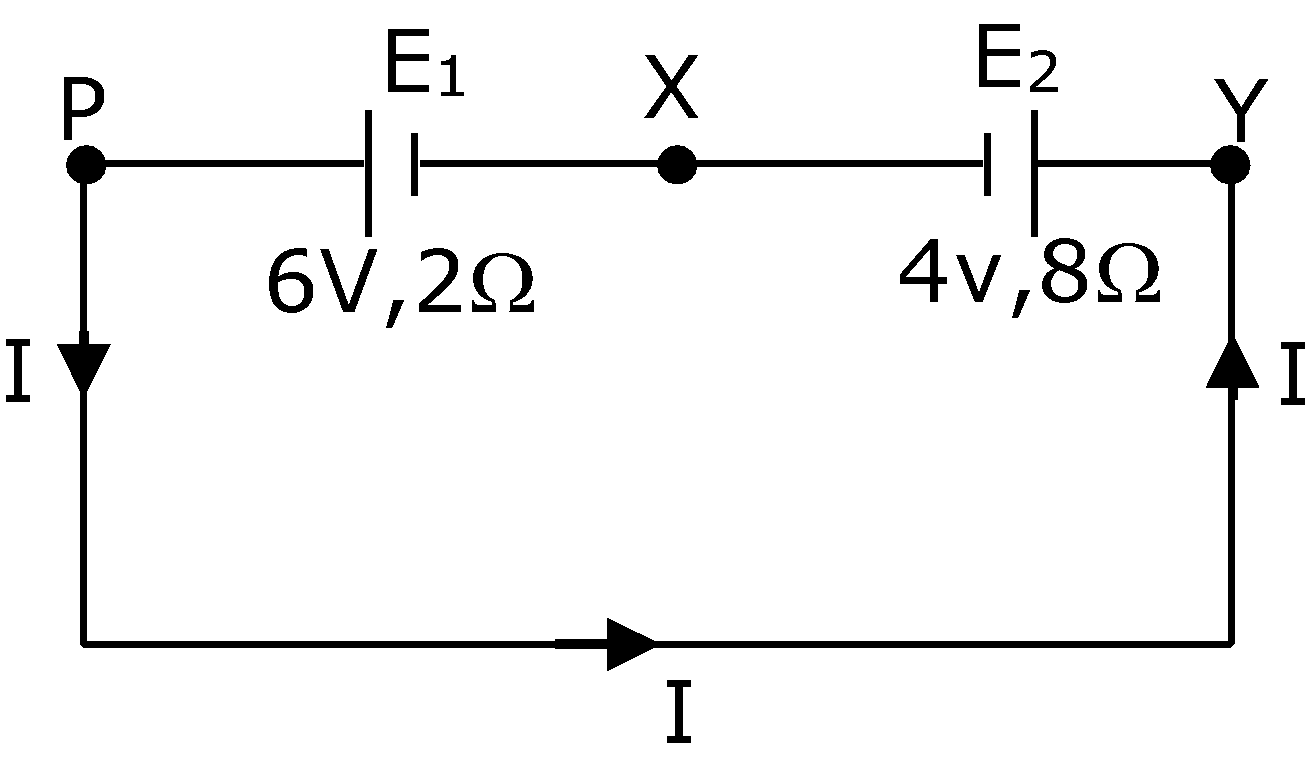# A cell E1 of emf 6V and internal resistance 2Ω is connected with another cell E2 of emf 4V and internal resistance 8Ω (as shown in the figure). The potential difference across points X and Y isa. 3.6V

b. 10.0V

c. 5.6V

d. 2.0Vemf of E1 = 6V

r1 = 2 Ω

emf of E2= 4V

r2 = 8Ω

|vx– vy| = potential difference across points X and Y

Eeff = 6 – 4 = 2V

Req = 2 + 8 = 10 Ω

So, current in the circuit will be

⇒ Ι = Eeff / Req⇒ I = 2/10 = 0.2 A

Now, potential difference across points X and Y is

|vx– vy| = E + iR

⇒ |vx– vy| = 4 + 0.2 × 8 = 5.6 V

⇒ |vx– vy| = 5.6 V(0)(0)